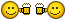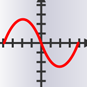Direction of PolylinesRecommended PostsI think i fix it . Thank you All

here is the code

(defun c:pld (/ ss i e j p a h)
(if
(setq ss (ssget '((0 . "*POLYLINE,SPLINE"))))
(progn
(setq h (* 0.05 (getvar 'viewsize)))
(repeat (setq i (sslength ss))
(setq e (ssname ss (setq i (1- i))))
(repeat (setq j (fix (vlax-curve-getendparam e)))
(setq j (1- j)
p (vlax-curve-getpointatparam e (+ j 0.5))
a ((lambda (d) (atan (cadr d) (car d))) (vlax-curve-getfirstderiv e (+ j 0.5)))
)
(grdraw p (polar p (+ a (* pi 0.9)) h) 3)
(grdraw p (polar p (- a (* pi 0.9)) h) 1)
)
)
)
)
(princ)
)

Thank you Stefan BMR for the code• Replies 52
• Created

Top Posters In This Topic

•5

•9

•23

•7

Top Posters In This Topic

•motee-z 5 posts

•marko_ribar 9 posts

•prodromosm 23 posts

•Stefan BMR 7 posts

Posted Imagesprodromosm,

Here is a version that works on spline too. Splines parametrization is quite different than polylines, so I decided to draw 10 arrows per spline.

I've added the color feature, depending of curve direction CW/CCW.

As I expected, these changes have resulted in a much longer code. And all of this just for a temporary arrow...

Don't get me wrong, I enjoy writing it and I was quite excited when I figure out how to find the direction of curve.

So let me ask you: what you need this for? I assume that you take an action or another, depending on curve direction.

So I can imagine that you can skip this intermediate step, but this is up to you.

This version is still designed just for objects in WCS.

It uses 3 color: white for open or self-intersecting curve; green for CW curve; red for CCW curve.

clockwise-p function doesn't work in Acad2011 (old pacman face is laughing at me).

I've tested it successfully in acad2012 only.

(defun c:test (/ *error* VxV clockwise-p acDoc ms ss i e j p a c h s f r d)
ms (if
(eq (getvar 'cvport) 1)
(vla-get-paperspace acDoc)
(vla-get-modelspace acDoc)
)
)
(vla-startundomark acDoc)

(defun *error* (m)
(and m (not (wcmatch (strcase m) "*CANCEL*,*EXIT*,*QUIT*")) (princ (strcat "\nError: " m)))
(vla-endundomark acDoc)
(princ)
)

(defun VxV (a b)
)
)
;(clockwise-p ename)                         ;
;argument:                                   ;
;   e - ename of curve                       ;
;       closed 2D curve in WCS               ;
;return: T for clockwise curve               ;
;----the pacman shape (and probably more)    ;
;    doesn't work in acad2011                ;
;A curve has the same orientation as an      ;
;infinitesimal segment positioned around its ;
;minima or maxima                            ;
;Stefan M. 11.11.2013                        ;
(defun clockwise-p (e / p1 p2 p a b d f1 f2)
(vla-getboundingbox (vlax-ename->vla-object e) 'p1 'p2)
(setq p  (vlax-curve-getparamatpoint
e
(vlax-curve-getclosestpointtoprojection
e
(vlax-safearray->list p1)
'(1 0 0)
)
)
a  (vlax-curve-getstartparam e)
b  (vlax-curve-getendparam e)
d  (if
(eq (cdr (assoc 0 (entget e))) "SPLINE")
(* 0.01 (- b a))
0.1
)
f1 (vlax-curve-getfirstderiv e (+ a (rem (+ p d) (- b a))))
f2 (vlax-curve-getfirstderiv e (+ a (rem (+ (- p d) (- b a)) (- b a))))
)
)
;selfinters[ecting object]                   ;
;argument:                                   ;
;   o - vla-object                           ;
;       CLOSED 2D curve in WCS               ;
;return: T for selfintersecting curve        ;
;Stefan M. 11.11.2013                        ;
(defun selfinters (o / a)
(or
(vl-catch-all-error-p
(setq a (vl-catch-all-apply 'vlax-invoke (list ms 'addregion (list o))))
)
(vla-delete (car a))
)
)

(if
(setq ss (ssget '((0 . "*POLYLINE,SPLINE"))))
(progn
(setq h (* 0.05 (getvar 'viewsize)))
(repeat (setq i (sslength ss))
(setq e (ssname ss (setq i (1- i)))
c (cond ((and                         ;arrows color
(vlax-curve-isplanar e)
(vlax-curve-isclosed e)
(not (selfinters (vlax-ename->vla-object e)))
)
(if (clockwise-p e) 3 1)     ;green if clocwise, red if not
)
(7)                 ;white for open, 3D, or selfintersecting curve
)
)
(if
(eq (cdr (assoc 0 (entget e))) "SPLINE")
(setq s (vlax-curve-getstartparam e)      ;start curve
f (vlax-curve-getendparam e)
j 10                     ;10 arrows on a single spline
d (/ (- f s) j)                     ;segment "length" (in paramter units)
)
(setq s 0.0
j (fix (vlax-curve-getendparam e))  ;1 arrow per segment for polylines
d 1.0
)
)
(repeat j
(setq j (1- j)
r (+ s (* j d) (* 0.5 d))           ;current parameter
p (vlax-curve-getpointatparam e r)
a ((lambda (d) (atan (cadr d) (car d))) (vlax-curve-getfirstderiv e r))
)
(grdraw p (polar p (+ a (* pi 0.9)) h) c)
(grdraw p (polar p (- a (* pi 0.9)) h) c)
)
)
)
)
(vla-endundomark acDoc)
(princ)
)

very nice stefan thank you very much

Thank you motee-z. You are welcome

Share on other sitesThank you Stefan BMR nice job

Can you use the red and green color and for the open polynes

from right ---> left green

from left ---> right red

Share on other sitesSo let me ask you: what you need this for?

I try to explain you why i need this this.

I use a lisp fot dimension polylines (open or close)

If the polyline is ccw the dimension text writes on top of the polyline (so red color)

if the polyline is cw the dimension text writes under the polyline (so green color)

So Stefan BMR can you add some lines code for the open polynes

from right ---> left (green)

from left ---> right (red)

Share on other sitesStephen, this is really impressive and useful.

The color coding works great and is very clear.

Thank you for sharing.

~Greg

Share on other sitesStephen, this is really impressive and useful.

The color coding works great and is very clear.

Thank you for sharing.

~Greg

Thank you Greg. I'm glad you find it useful.

This

(vlax-curve-getparamatpoint e
(vlax-curve-getclosestpointtoprojection e
(vlax-safearray->list p1)
'(1 0 0)
)
)

should be

(vlax-curve-getparamatpoint e
(vlax-curve-getclosestpointtoprojection e
[color=red] (mapcar '- [/color](vlax-safearray->list p1)[color=red] '(1 1 0))[/color]
'(1 0 0)
)
)

I try to explain you why i need this this.

I use a lisp fot dimension polylines (open or close)

If the polyline is ccw the dimension text writes on top of the polyline (so red color)

if the polyline is cw the dimension text writes under the polyline (so green color)

So Stefan BMR can you add some lines code for the open polynes

from right ---> left (green)

from left ---> right (red)

It looks simple, but there are numerous "exceptions".

What direction has a shape like this >?

I'd really like to see a sample from you, before and after, pline/spline, open/closed, CW/CWW in any combination...Share on other sitesShare on other sitesnice job The color coding works great. If you fix

from right ---> left green

from left ---> right red

will be fantastic !!Share on other sitesnice job The color coding works great. If you fix

from right ---> left green

from left ---> right red

will be fantastic !!Assuming e for polyline's ename

(setq start (vlax-curve-getstartpoint e)
end   (vlax-curve-getendpoint e))
(if (< (car start) (car end))
(princ "\nLeft to right"); or (setq color acRed)
(princ "\nRight to left"); or (setq color acGreen)
)

Share on other sitesEdited by prodromosm
Share on other sitesAssuming e for polyline's ename

(setq start (vlax-curve-getstartpoint e)
end   (vlax-curve-getendpoint e))
(if (< (car start) (car end))
(princ "\nLeft to right"); or (setq color acRed)
(princ "\nRight to left"); or (setq color acGreen)
)

How can i use it ? in which line put the code?

Share on other sitesWhat you think is a simple "left->right" thing is in fact more a particular situation from many, many possibilities. I ask you to post a sample so we can judge all possibilities and to write a solid code that will cover them all.

Because, sooner or later, you will run into an "exception" and you will come here and ask us to make a little modification, then tomorrow again, and again...

About your last question... Seriously, man? I thought it is really simple.

Just replace

(7)  ;white....

with

((if (< (car (vlax-curve-getstartpoint e)) (car (vlax-curve-getendpoint e))) 1 3))

Note the double brackets.

Share on other sitesthank you Stefan BMRJoin the conversation

You can post now and register later. If you have an account, sign in now to post with your account.
Note: Your post will require moderator approval before it will be visible.×   Pasted as rich text.   Restore formatting

Only 75 emoji are allowed.